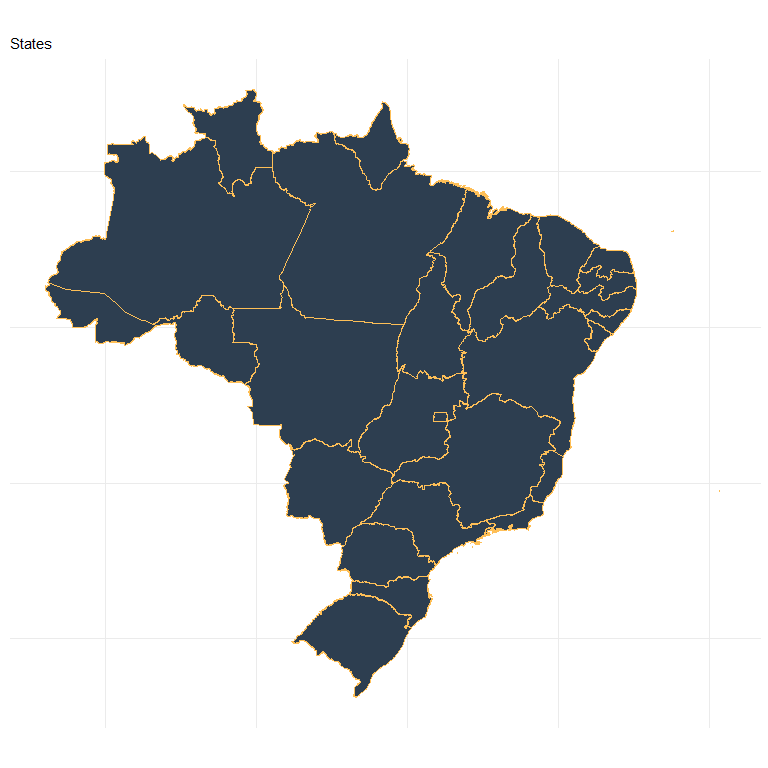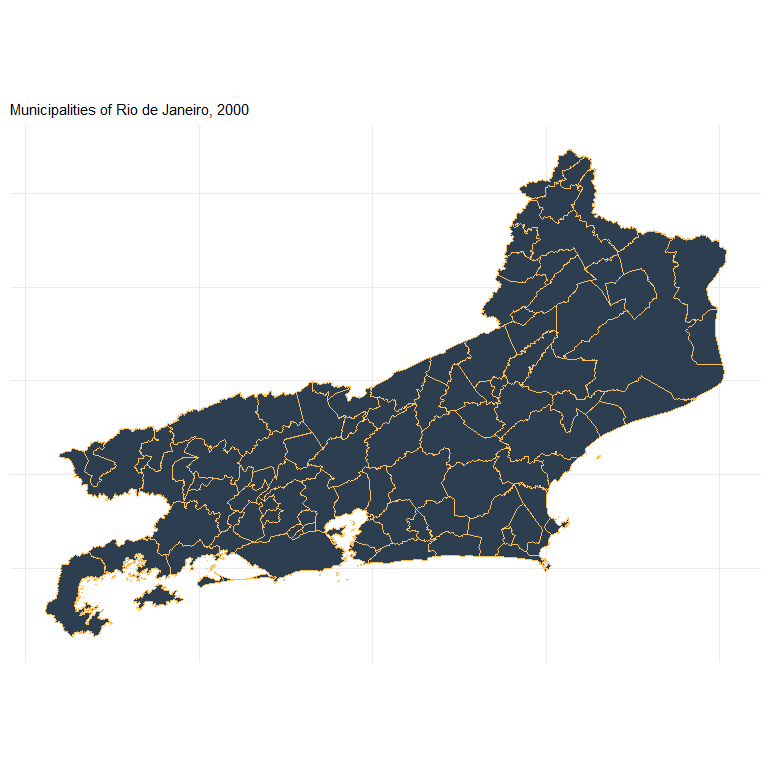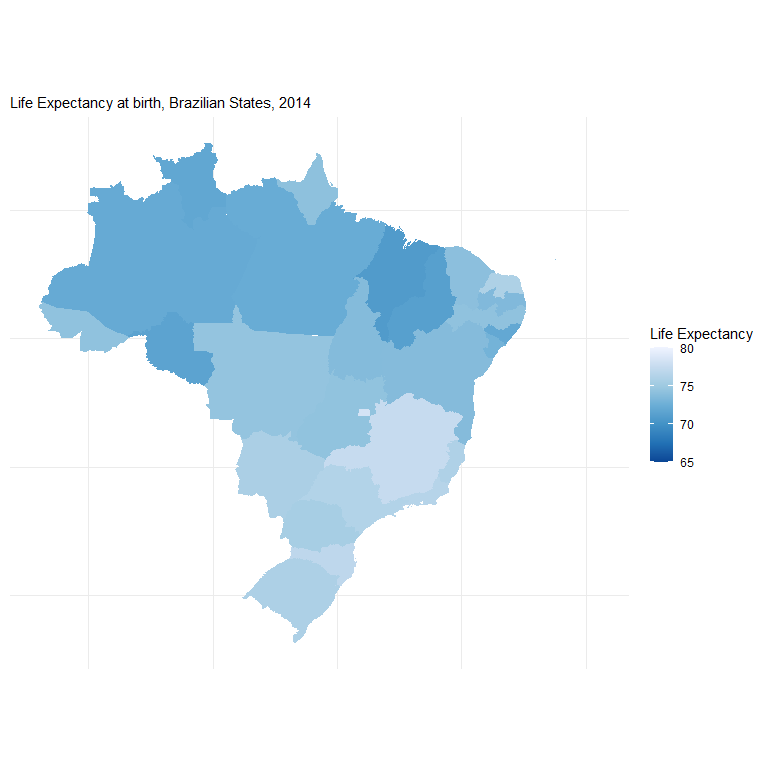# Introduction to geobr

The geobr package provides quick and easy access to official spatial data sets of Brazil. The syntax of all geobr functions operate on a simple logic that allows users to easily download a wide variety of data sets with updated geometries and harmonized attributes and geographic projections across geographies and years. This vignette presents a quick intro to geobr.

## Installation

You can install geobr from CRAN or the development version to use the latest features.

# From CRAN
install.packages("geobr")

# Development version
utils::remove.packages('geobr')
devtools::install_github("ipeaGIT/geobr", subdir = "r-package")

Now let’s load the libraries we’ll use in this vignette.

  library(geobr)
library(ggplot2)
library(sf)
library(dplyr)

## General usage

### Available data sets

The geobr package covers 21 spatial data sets, including a variety of political-administrative and statistical areas used in Brazil. You can view what data sets are available using the list_geobr() function.

# Available data sets
datasets <- list_geobr()

print(datasets, n=21)

## Download spatial data as sf objects

The syntax of all geobr functions operate one the same logic, so the code to download the data becomes intuitive for the user. Here are a few examples.

# State of Sergige

# Municipality of Sao Paulo
muni <- read_municipality( code_muni = 3550308, year=2010 )

# All municipalities in the state of Alagoas

# All census tracts in the state of Rio de Janeiro
cntr <- read_census_tract(code_tract = "RJ", year = 2010)

If the parameter code_ is not passed to the function, geobr returns the data for the whole country by default.

meso <- read_intermediate_region(year=2017)
states <- read_state(year=2019)

## Important note about data resolution

All functions to download polygon data such as states, municipalities etc. have a simplified argument. When simplified = FALSE, geobr will return the original data set with high resolution at detailed geographic scale (see documentation). By default, however, simplified = TRUE and geobr returns data set geometries with simplified borders to improve speed of downloading and plotting the data.

## Plot the data

Once you’ve downloaded the data, it is really simple to plot maps using ggplot2.

# Remove plot axis
no_axis <- theme(axis.title=element_blank(),
axis.text=element_blank(),
axis.ticks=element_blank())

# Plot all Brazilian states
ggplot() +
geom_sf(data=states, fill="#2D3E50", color="#FEBF57", size=.15, show.legend = FALSE) +
labs(subtitle="States", size=8) +
theme_minimal() +
no_axisPlot all the municipalities of a particular state, such as Rio de Janeiro:


library(ggplot2)

all_muni <- read_municipality( code_muni = "RJ", year= 2010)

# plot
ggplot() +
geom_sf(data=all_muni, fill="#2D3E50", color="#FEBF57", size=.15, show.legend = FALSE) +
labs(subtitle="Municipalities of Rio de Janeiro, 2000", size=8) +
theme_minimal() +
no_axis## Thematic maps

The next step is to combine data from geobr package with other data sets to create thematic maps. In this example, we will be using data from the (Atlas of Human Development (a project of our colleagues at Ipea))[https://atlasbrasil.org.br/] to create a choropleth map showing the spatial variation of Life Expectancy at birth across Brazilian states.

#### Merge external data

First, we need a data.frame with estimates of Life Expectancy and merge it to our spatial database. The two-digit abbreviation of state name is our key column to join these two databases.

# Read data.frame with life expectancy data
df <- utils::read.csv(system.file("extdata/br_states_lifexpect2017.csv", package = "geobr"), encoding = "UTF-8")

states$name_state <- tolower(states$name_state)
df$uf <- tolower(df$uf)

# join the databases
states <- dplyr::left_join(states, df, by = c("name_state" = "uf"))

#### Plot thematic map

  ggplot() +
geom_sf(data=states, aes(fill=ESPVIDA2017), color= NA, size=.15) +
labs(subtitle="Life Expectancy at birth, Brazilian States, 2014", size=8) +
scale_fill_distiller(palette = "Blues", name="Life Expectancy", limits = c(65,80)) +
theme_minimal() +
no_axis